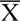Check out some great books and help the site! I have chosen these books as among the best to illustrate this subject.

# Roman Units of Measurement

*Special thanks to Richard Campbell for his help here.

## Roman Length Measurements

 1 uncia = 24.6 mm/0.97 inch Roman inch 12 unciae = 1 pes = 295.7 mm/11.6 inches Roman foot 1.5 pedes = 1 cubitum = 2.5 pedes = 1 gradus = 2 gradus = 1 passus = 1.48 m/1.62 yards Roman pace 125 passus = 1 stadium = 1000 passus = 1 mille (mille passus) = 1480 m/1618 yards Roman mile

## Liquid Measurements

 The standard unit in liquid measurements was the sextarius, which was about the equivalent of one English pint (0.568 litre). 4 quartarii = 1 sextarius 6 sextarii = 1 congius 4 congii = 1 urna 2 urnae = 1 amphora

## Roman Months

The Roman year revolved around the agricultural calendar, so as the sowing period started in March, this was traditionally the start of the year..

In winter there was less activity in farming than in summers, so these months were not actually named. They were considered the dead months. It was not until later in the Roman Empire that the whole of the year was standardised into the format we know and still use today.

 January = Januarius February = Februarius March = Martius April = Aprilis May = Maius June = Junius July = Julius August = Augustus September = September October = October November = November December = December

## Roman Area Measurements

 The basic measurement in Roman agricuture was the iugerium which was approximately 3000 square yards. This was reckoned to be the area of land a pair of oxen could plough in a day.. 1 iugerium 2 iugeria = 1 heredium 100 heredia = 1 centuria

## Roman Numerals

 I = unus = 1 II = duo = 2 III = tres = 3 IV = quattor = 4 V = quinque = 5 VI = sex = 6 VII = septem = 7 VIII = octo = 8 IX = novem = 9 X = decem = 10 XX = viginti = 20 XXX = triginta = 30 XL = quadriginta = 40 L = quinquaginta = 50 C = centum = 100 CC = ducenti = 200 CCC = trecenti = 300 CD = quadringenti = 400 D = quingenti = 500 M = mille = 1000=written with a bar above them, numbers are multiplied by 1'000, so this:=10,000= written with a bar above with tails descending, numbers are multiplied by 100,000, so this:=1,000,000 *Thanks to Richard Campbell for loaning me the book that made this clear.Romans in Britain
www.romanobritain.org